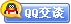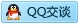##用户名 Email 自动登录 找回密码 密码 会员注册
 VIP会员，3年作业免费下 ！ 奥鹏作业，奥鹏毕业论文检测 新手作业下载教程，充值问题 没有找到答案，请在此处留言！ 2020年04月最新全国统考资料 投诉建议，加盟合作！奥鹏课程积分软件(ver:3.1)

# 东大11春学期《C语言及程序设计》在线作业一（免费）发表于 2011-7-1 10:50:05 | 显示全部楼层 |阅读模式东北大学 11春学期《C语言及程序设计》在线作业一 单选题 1.若有定义：intx=7;floata=2.5,b=4.7;则表达式a+x%3*(int)(a+b)%2/4的值是（）。 A. 2.5 B. 2.75 C. 3.5 D. 0 答案：A 2.以下是正确的C语言标识符是（）。 A. #define B. _123 C. %d D. \n 答案：B 3.下列四组选项中，均不是C语言关键字的选项是()。 A. defineIFtype B. getccharprintf C. includescanfcase D. whilegopow 答案：A 4.合法的C语言标识符是（）。 A. abc B. 2ab C. _a1 D. /n 答案：C 5.下列四组选项中，均是不合法的用户标识符的选项是______。 A. AP_0do B. floatgoto_A C. b-agotoint D. _123tempINT 答案：C 6.执行下列语句后，第一个输出的值为（）#includestdio.h;#defineM;#defineN;M+1;main(){printf(“%d\n”,N);} A. 3 B. 4 C. 6 D. 8 答案：A 7.若运行以下程序时，从键盘输入25,13,10回车，则输出结果为（）。main(){inta1,a2,a3;scanf("%d%d%d",a1,a2,a3);printf("a1+a2+a3=%d\n",a1+a2+a3);} A. a1+a2+a3=48 B. a1+a2+a3=25 C. a1+a2+a3=10 D. 不确定值 答案：D 8.下面四个选项中，均是合法转义字符的选项是（）。 A. \"""\\""\n" B. "\""\017""\"""" C. \018""\f""xab" D. \\0""\101""xlf" 答案：A 9.若有以下定义：intm=7,n=12；则能得到值为3的表达式是（）。 A. n%=(m%=5) B. n%=(m-m%5) C. n%=m-m%5 D. (n%=m)-(m%=5) 答案：D 10.设int类型的数据长度为2个字节，则unsignedint类型数据的取值范围是（）。 A. 0～255 B. 0～65535 C. -32768～+32767 D. -256～+255 答案：B 11.071^052的结果是（） A. 32 B. 16 C. 23 D. 22 答案：A 12.下列程序的输出为（）#includestdio.h;main(){intm=13;floata=12.6,x;x=m/2+a/2;printf(“%f\n”,x);} A. 12 B. 12.3 C. 12.8 D. 12.3 答案：B 13.下面四个选项中，均是C语言关键字的选项是（）。 A. autoenuminclude B. switchtypedefcontinue C. signedunionscanf D. ifstructtype 答案：B 14.若有变量说明：inta=0,b=0,c=0;，以下符合C语言语法的赋值表达式是（）。 A. a=9+b+c=a+9 B. a=9+b;c=a+9; C. "a=(9+b,b++)" D. a=9+b++=a+7 答案：C 15.下面程序的输出是（）。main(){intx=10,y=3;printf(“%d\n”,y=x%y);} A. 0 B. 1 C. 3 D. 不确定的值 答案：B 判断题 1.在C程序中，逗号运算符的优先级最低 A. 错误 B. 正确 答案：B 2.一个C源程序可由一个或多个函数组成 A. 错误 B. 正确 答案：B 3.当从键盘输入数据时，对于整型变量只能输入整型数值，对于实型变量只能输入实型数值 A. 错误 B. 正确 答案：A 4.在C程序中main函数必须位于程序的最前面 A. 错误 B. 正确 答案：A 5.C语言本身没有输入输出语句 A. 错误 B. 正确 答案：B 久爱奥鹏网：www.92open.com
 本版积分规则 回帖并转播 回帖后跳转到最后一页客服一客服二客服三客服四微信客服扫一扫